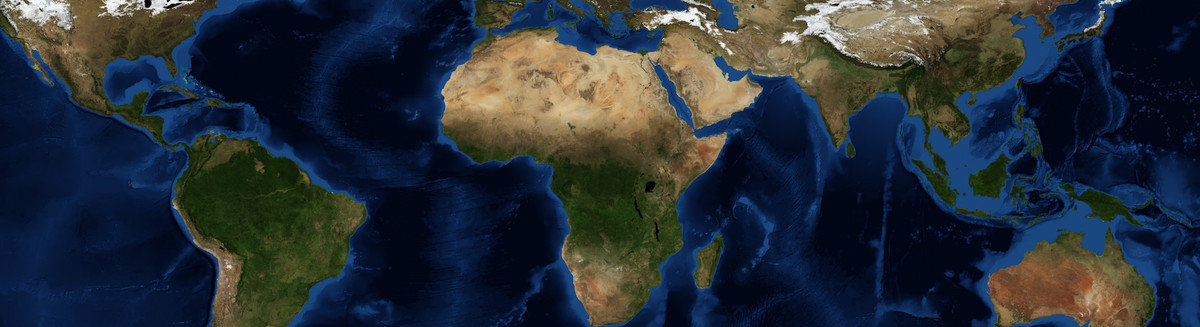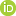This is a Preprint and has not been peer reviewed. This is version 2 of this Preprint.

##### Downloads

Download Preprint

##### Authors

Ernesto Rodriguez, Michael Durand, Renato Prata de Moraes Frasson

##### Abstract

The NASA/CNES Surface Water and Ocean Topography (SWOT) mission will estimate global river discharge using remote sensing. Synoptic remote sensing data extends in situ point measurements, but, at any given point, is generally less accurate. We address two questions: 1)What are the scales at which river dynamics can be observed, given spatial sampling and measurement noise characteristics? 2) Is there an equation whose variables are the averaged hydraulic quantities obtained by remote sensing, and which describes the dynamics of spatially averaged rivers? We use calibrated hydraulic models to examine the power spectra of the different terms in the momentum equation, and conclude that the measurement of river slope sets the scale at which rivers can be observed. We introduce the reach-averaged Saint-Venant equations, that involve only observable hydraulic variations, and which parametrize within-reach variability with a variability index that multiplies the friction coefficient and leads to an increased ”effective” friction coefficient. An exact expression is derived for the increase in the effective friction coefficient, and we propose an approximation that requires only estimates of the hydraulic parameter variances. We validate the results using a large set of hydraulic models and find the approximated variability index is most faithful when the river parameters obey lognormal statistics. The effective friction coefficient, which can vary from a few percent to more than 50% of the point friction coefficient, is proportional to the river bed elevation variance and inversely proportional to the depth. This has significant implications for estimating discharge from SWOT data.

##### DOI

https://doi.org/10.31223/osf.io/euqmx

##### Subjects

Earth Sciences, Hydrology, Physical Sciences and Mathematics

##### Keywords

remote sensing, upscaling, hydrology, Manning coefficient, River discharge, Saint-Venant Equations, SWOT

##### Dates

Published: 2019-10-30 21:01

##### License

CC BY Attribution 4.0 International

Add a Comment

You must log in to post a comment.

Comments

There are no comments or no comments have been made public for this article.